# Linear Function

## Linear Function

Linear Function is defined as the real valued function $f : R \rightarrow R$ , y = f(x) = mx + c for each $x \in R$ where m and c is a constant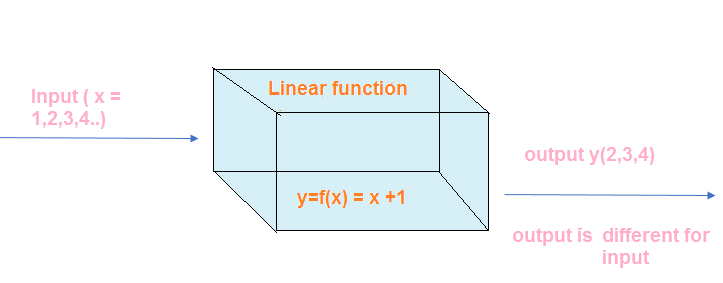$y = x+ 2$
$y=2x -3$
$y=x-2$
$y =4x$
The above all are example of linear function

## Domain and Range of the Linear Function

For $f : R \rightarrow R$ , y = f(x) = mx + c for each $x \in R$

Domain = R
Range = R

## Graph of the Linear Function

We can draw the Linear Function graph on the Cartesian plane with value of x on the x-axis and value of y=f(x) on the y-axis. We can plot the point and join the point to obtain the graph. The graph of the linear function is a straight line

Steps to Draw the Graph of Linear Function
For $y = f(x) = mx + c$
1. Find the value of y for x=0
y=c
This point will lie on Y -axis. And the coordinates will be (0,c)
2. Find the value of x for y=0
$x= -\frac {c}{m}$
This point will lie on X -axis. And the coordinates will be $(-\frac {c}{m}, 0)$
3. This can be represent in below linear table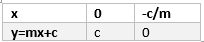4. Now we can draw the line joining these two point
5. We can take any other convienent points also to draw the line

For $y = f(x) = mx + c$
$m$ is the slope and $c$ is the $y$ intercept of the graph.
If $m$ is positive
then the line rises to the right and if $m$ is negative then the line falls to the right
Below are few graph based on values of m and c
Graph for the linear function with m and c both positive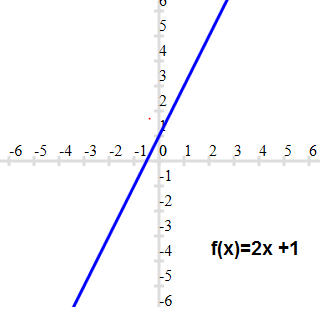Graph for the linear function with positive m and negative c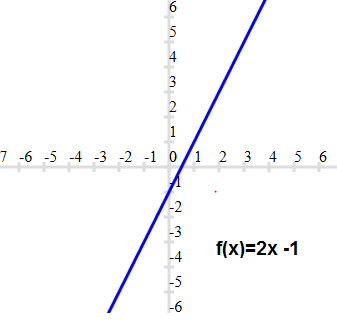Graph for the linear function with m and c both negative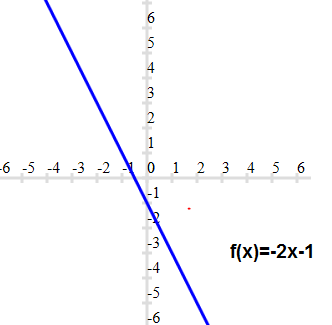Graph for the linear function with negative m and positive c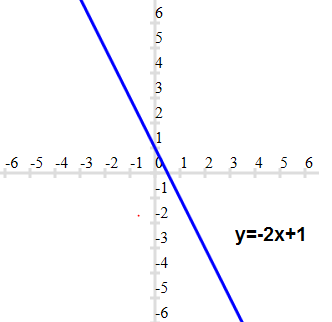Graph for the linear function with positive m and c=0. This passes through origin. Such type of linear function is also called proportional function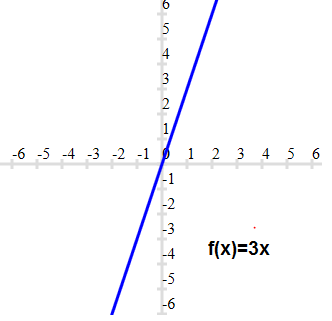Graph for the linear function with negative m and c=0. This passes through origin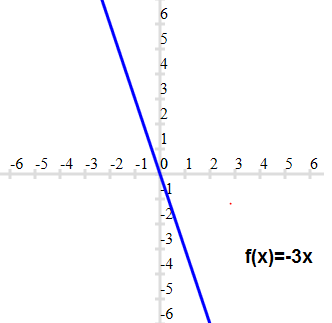Identify Function and constant function are special cases of Linear function
if m =1 and c=0, Linear function becomes f(x) =x which is a Identity Function
If m=0 ,then Linear function becomes f(x) =c which is a Constant Function

## Solved examples of Linear Functions

1. which is below function is a Linear function?
a. $y =2x$
b. $y = 11 -x$
c. $y= \frac {2}{3} x + \frac {1}{4}$
d. $x^2 + y^2=1$
e. $y =x^3$
f. $y =x^2 +1$
Solution
For the function to be a Linear function ,it should be of the form (mx+c)
a. This is Linear function as of the form (mx+c)
b. This is Linear function as of the form (mx+c)
c. This is Linear function as of the form (mx+c)
d. This is not a linear function
e. This is not a linear function
f. This is not a linear function

2. which of the graph represent Linear function?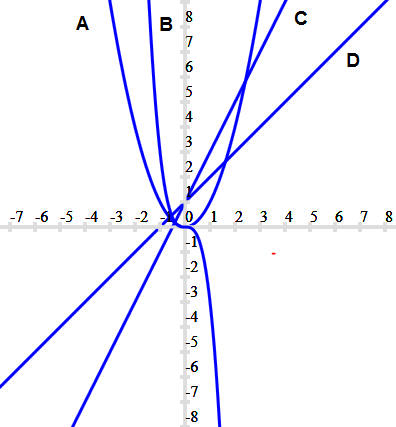Solution
The graph should be straight line for the function to be Linear function
So C and D are Linear function

3. Let f = {(1,1), (2,3), (0, -1), (-1, -3)} be a linear function from Z into Z.
Find f(x).
Solution
Since f is a linear function, f (x) = mx + c. Also, since $(1, 1), (0, - 1) \in f$,
f (1) = m + c = 1 and f (0) = c = -1. This gives m = 2 and so,f(x) = 2x - 1.

### Quiz Time

Question 1.
The function 't' which maps temperature in degree Celsius into temperature in degree Fahrenheit is defined by
$t(C) =\frac {9}{5} C + 32$
Which of the following is incorrect?
A.t(0)=32
B. t(-5)=23
C. t(10)=48
D. None of the above
Question 2.
If a function is defined as $f = {(x, y) | y = 2x + 7, \; where \; x \in R \; and \; -5 \leq x \leq 5}$ is a relation. Then find the domain and Range of Function?
A. Domain=[-5,5], range=[3,17]
B. Domain=[-5,5], range=[-3,17]
C. Domain=[-5,5], range=[-3,-17]
D. Domain=[-5,5], range=[-5,5]
Question 3.
Let f = {(1,1), (2,3), (3,5), (4,7)} be a linear function from Z into Z.
and f(x) =px +q then
A. p=1,q=1
B. p=1,q=2
C. p=2,q=1
D. p=2,q=-1
Question 4.
Let $f(x) =c$,find the value of $f(2) -f(1)$
A. 4
B. 2
C. 0
D. 1
Question 5.
The slope of the Linear function y=11x-1 is
A. 0
B. 11
C. -1
D. None of these
Question 6.
Find the Range and domain of the function $f(x) =x +2$
A. Domain = R, Range =R
B. Domain = R - {2}, Range = R
C. Domain = R , Range = R -{2}
D. Domain = R - {1}, Range = R